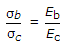# Civil Engineering - Theory of Structures

### Exercise :: Theory of Structures - Section 4

11.

The load on a spring per unit deflection, is called

 A. stiffness B. proof resilience C. proof stress D. proof load.

Explanation:

No answer description available for this question. Let us discuss.

12.

In a shaft, the shear stress is not directly proportional to

 A. radius of the shaft B. angle of twist C. length of the shaft D. modulus of rigidity.

Explanation:

No answer description available for this question. Let us discuss.

13.

Ab and Ac are the cross sections of bronze and copper bars of equal length, σb, σc are their respective stresses due to load P. If Pb and Pc are the loads shared by them, (where Eb and Ec are their modulii).

 A.B. P = Pb + Pc C. P = Ab σb + Ac σb D. all the above

Explanation:

No answer description available for this question. Let us discuss.

14.

A compound truss may be formed by connecting two simple rigid frames, by

 A. two bars B. three bars C. three parallel bars D. three bars intersecting at a point.

Explanation:

No answer description available for this question. Let us discuss.

15.

The locus of the end point of the resultant of the normal and tangential components of the stress on an inclined plane, is

 A. circle B. parabola C. ellipse D. straight line.